# Micro Servo (0.12s / 1.2kg-cm - 9g SG90)

The micro Servo motor is a small motor which turns up to 180 degrees or ranges between 90, 180, or 270 degrees depending on the make or type, but never 360.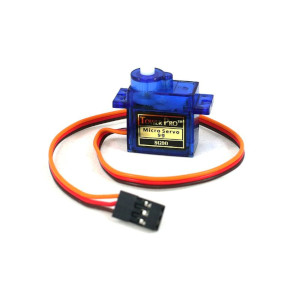## How it works​

The Servo motor is an electromechanical device that rotates and changes movement based on the supplied current and voltage. The Servo works as a closed loop system that provides torque and velocity as commanded from its controller. This allows the servo to receive feedback to know when to close the loop. When connected to a programmable circuit board, this device uses the Pulse Width Modulation pins.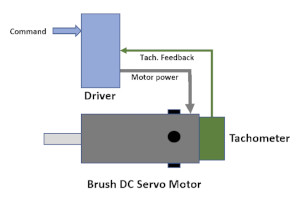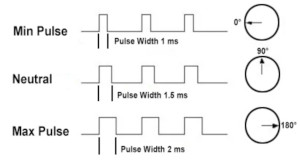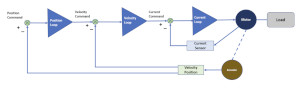## Wiring​

• Red Wire: connected to 5V
• Black Wire: connected to ground
• Brown Wire: connect to a PWM pin (#~)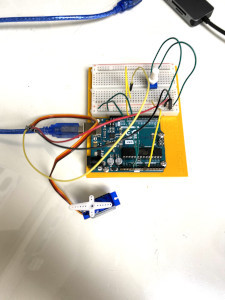## Wiring Guide​

• Connect the voltage and ground wires to the appropriate connection to the Servo
• Connect the signal wire of the Servo to a PWM pin in the digital input pin section of the Arduino board
• Connect the terminal pins of the potentiometer to voltage and ground
• Connect the wiper pin of the potentiometer to an analog pin
• Follow the code provided and observe how the servo rotates as you change the values of the potentiometer

## Programming​

The following code demonstrates how you can move the servo motor by mapping the potentiometer. If you want to skip the steps, you can jump to the Full Code.

Step 1: Create variables for the sensors pin and a variable to store the data that is collected

``int sensor = A0; //sensor pin connection``

Step 2: Set up serial communication, set the sensor pin as an INPUT.

``void setup() { Serial.begin(9600); // start the serial port pinMode(sensor, INPUT); //setting the pin to the Arduino}``

Step 3: Read the data from the sensor, and convert the data into a volts measurement

``void loop() { //5V float volts = analogRead(sensor)*0.0048828125;  // value from sensor * (5/1024)``

Step 4: Now convert the volts measurement into distance, a measurement we understand. This calculation is found in the datasheet gragh of the component and can be found here!

``int distance = 13*pow(volts, -1); // worked out from datasheet graph delay(1000); // slow down serial port ``

Step 5: Finally use the distance we calculated and display ONLY when the distance is less than or equal to its max limit.

``if (distance <= 30){   Serial.println(distance);   // prints the distance }}``

## Full Code​

``#include <Servo.h>Servo servo;  // Servo objectint servoPin = 9;int potpin = 0;  // analog pin used to connect the potentiometerint val;    // variable to read the value from the analog pinvoid setup() { servo.attach(servoPin);  // attaches the servo on pin 9 to the servo object}void loop() { val = analogRead(potpin);            // reads the value of the potentiometer (value between 0 and 1023) val = map(val, 0, 1023, 0, 180);     // scales the values to use with the servo (value between 0 and 180) servo.write(val);                  // sets the servo position according to the scaled value delay(15);                         }``

## Output​

Serial Monitor will display distance that is measure from the proximity sensor, only if the distance measure is less than or equal to the max limit which is 30cm for this exact component.